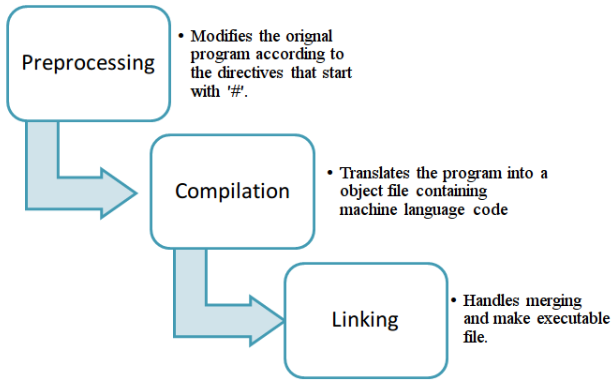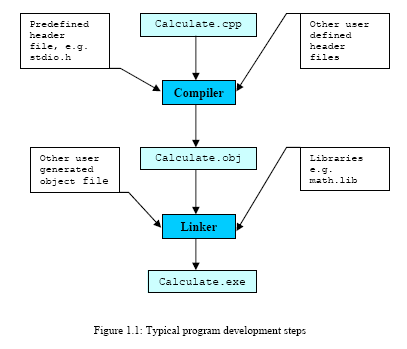# Haste makes waste1. 预编译，将带有预编译符号比如#include #define的代码，变成纯C++代码(不含预编译符的)
2. 编译：将上面预编译之后的输出处理成obj文件，这个文件机器能够识别。
3. 链接：将上面编译后的所有obj文件组合起来，生成lib或是可执行的exe文件等。

In summary to compile in a terminal:

• Open a terminal window
• change the working directory to the directory of the program
• Make sure names of folders and files do not have spaces in them
• To compile the program: g++ filename.cpp -o executableName
• To execute the program: ./executableName

Common mistakes when executing in the terminal:

• Make sure there are no spaces in filenames
• Make sure all the files you need are in the working directory (including header files), use ‘ls’ to check
``````#include <iostream>
#include <stdio.h>

int main()
{
int userInput = 0;
int maxNumber = 0;
int minNumber = 100;
int sumTotal = 0;
float average = 0;

//get the numbers from the user
for(int i = 0; i < 15; i++)
{
std::cout << "Enter a number: ";
scanf("%d", &userInput);
std::cout << userInput << "\n";

if(userInput > maxNumber)
{//the biggest number entered so far is the max number
maxNumber = userInput;
}
if(userInput < minNumber)
{//the lowest number entered so far is the min number
minNumber = userInput;
}
sumTotal = sumTotal + userInput;
}
std::cout << "Maximum number = " << maxNumber << "\n";
std::cout << "Minimum number = " << minNumber << "\n";
average = sumTotal / 15;
std::cout << "Average = " << average << "\n";
return 0;
}
``````
``````Enter a number: 123
Enter a number: 2
Enter a number: 34
Enter a number: 6
Enter a number: 57
Enter a number: 9
Enter a number: 876
Enter a number: 90
Enter a number: 11
Enter a number: 23
Enter a number: 876
Enter a number: 4
Enter a number: 13
Enter a number: 87
``````